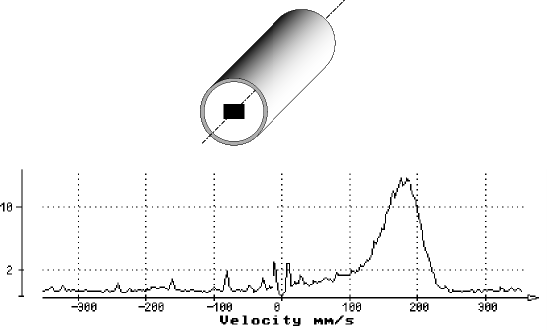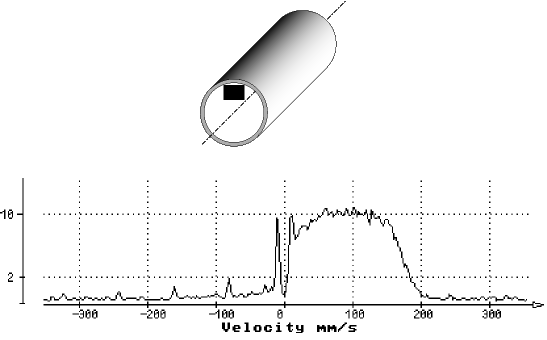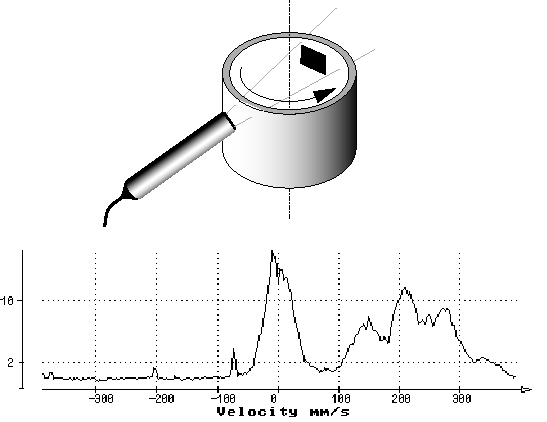# Using the power spectrum (FFT) of echoes coming from one gate

## Why using the power spectrum of one gate

The DOP can compute the power spectrum of a data series by means of an FFT algorithm. The data series is formed by samples taken on the demodulated I and Q signals for a selected depth which corresponds to one gate.

Before the computation of the power spectrum, the data series is filtered by a high pass filter which removes all the stationary components contained in the demodulated signals. In order to make the reading of the frequency scale more easy, the frequency scale is converted in velocity by using the standard Doppler formula. The ordinate gives the relative amplitude, in a logarithmic scale, of the power spectrum.

The computation of power spectrum is the best way to analyze the frequency content of the signal issue from a gate. When once looks at the velocity profile, all the gates give a single value of velocity. These values are the result of a computation of the mean Doppler frequency, which is the real mean value (non biased) of the power spectrum. This means that no information is given about the distribution of the Doppler energy. The same value of velocity can result from many different frequency distributions. The display of the complete power spectrum is a good method to increase the knowledge on the measured velocity values.## How to use the information contained in the power spectrum

The following examples will display different situations where the power spectrum can increase the knowledge in the measured velocity values.

The first situation (see figure) shows the computed power spectrum of a gate placed in the middle of a tube where a liquid is flowing. As shown in the power spectrum the sampling volume does not contain a single Doppler frequency. The width of the peak is related to the number of Doppler frequencies present in the sampling volume.

In the second situation (see figure), the sampling gate has been moved closer to the wall of the tube. The width of the Doppler peak is now much larger which means that much more different velocities are present in the sampling volume.

The power spectrum reveals also a small influence of the movements of the walls. As the power spectrum is computed from the high-passed filtered data values the amplitude of the power spectrum at the origin is always zero.The third situation (see figure), considers a rotating cylinder filled of liquid. The ultrasonic beam crosses the cylinder perpendicularly to the axis of the cylinder. The sampling volume is placed inside the cylinder in a position near the wall.

The power spectrum reveals a very strong influence of the movements of the walls of the cylinder despite the sampling volume does not touch a wall. This situation may appear if some of the multiple ultrasonic reflections may coincide with the sampling time of the echo. In such a case the velocity value is corrupted by the Doppler effect induced by the movement of the walls. The display of the power spectrum indicates clearly that the mean Doppler frequency computed is in fact the result of the mean value of two different velocity components, one coming from the movements of the walls and the other coming from the movements of the particles contained in the liquid.